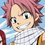# Quadratic equation + Trigonometry problem

If the quadratic equation $ax^2+bx+c=0$ has equal roots where a, b and c denote the lengths of the sides opposite to vertices A, B and C of a triangle ABC respectively, then find the sum of integers in the range of

$\frac{\sin A}{\sin C}+\frac{\sin C}{\sin A}$

I am stuck on this problem from quite some time and finally decided to post this here.

Since the roots are equal, I get $\sin^2B-4\sin A\sin C=0$. I thought of replacing B with $\pi-A-C$ and plug that in Wolfram Alpha but I get no nice solutions. I honestly don't know how to proceed in this problem.

Any help is appreciated. Thanks!Note by Pranav Arora
6 years, 9 months ago

This discussion board is a place to discuss our Daily Challenges and the math and science related to those challenges. Explanations are more than just a solution — they should explain the steps and thinking strategies that you used to obtain the solution. Comments should further the discussion of math and science.

When posting on Brilliant:

• Use the emojis to react to an explanation, whether you're congratulating a job well done , or just really confused .
• Ask specific questions about the challenge or the steps in somebody's explanation. Well-posed questions can add a lot to the discussion, but posting "I don't understand!" doesn't help anyone.
• Try to contribute something new to the discussion, whether it is an extension, generalization or other idea related to the challenge.

MarkdownAppears as
*italics* or _italics_ italics
**bold** or __bold__ bold
- bulleted- list
• bulleted
• list
1. numbered2. list
1. numbered
2. list
Note: you must add a full line of space before and after lists for them to show up correctly
paragraph 1paragraph 2

paragraph 1

paragraph 2

[example link](https://brilliant.org)example link
> This is a quote
This is a quote
    # I indented these lines
# 4 spaces, and now they show
# up as a code block.

print "hello world"
# I indented these lines
# 4 spaces, and now they show
# up as a code block.

print "hello world"
MathAppears as
Remember to wrap math in $$ ... $$ or $ ... $ to ensure proper formatting.
2 \times 3 $2 \times 3$
2^{34} $2^{34}$
a_{i-1} $a_{i-1}$
\frac{2}{3} $\frac{2}{3}$
\sqrt{2} $\sqrt{2}$
\sum_{i=1}^3 $\sum_{i=1}^3$
\sin \theta $\sin \theta$
\boxed{123} $\boxed{123}$

Sort by:

Let the circumradius of the triangle be $R$, so from the sine rule: $\frac{a}{\sin A}= \frac{b}{\sin B}= \frac{c}{\sin C}= 2R$ Then, note that $\frac{\sin A}{\sin C} + \frac{\sin C}{\sin A}= \frac{\frac{2R}{a}}{\frac{2R}{c}} + \frac{\frac{2R}{c}}{\frac{2R}{a}}= \frac{a}{c} + \frac{c}{a} = \frac{a^2+c^2}{ac}$
The roots of the given equation will be equal iff $b^2-4ac= 0$ From the cosine rule, we obtain $b^2= c^2 + a^2 - 2ac \cos (B)$ Hence, $c^2 + a^2 - 2ac \cos (B) - 4ac = 0$ $\implies c^2 + a^2 = ac(4 + 2 \cos (B) )$ $\implies \frac{c^2+a^2}{ac}= 4 + 2 \cos (B)$ From the inequalities (note that the inequalities are strict) $-1 < \cos (B) < 1$, we obtain: $4< \frac{c^2+a^2}{ac} < 6$

- 6 years, 9 months ago

Is the actual answer 12 ? I did nothing special, just denote given expression by E. and as we get from the condition of equal roots , replace your sin^2x, by (1-cos^2x) then write the expression for cosx for a triangle. you will obtain an eqn. like, [(E/2)-2]^2= 1-4ac which in turn gives the simple inequality, (E-2)(E-6)<0
since a and c both>0 i.e 2<E<6.(the lower limit is also obvious from A.M-G.M inequality, anyway,) Therefore the sum of the integers lying between this range is =(3+4+5)=12.(ans) SORRY FOR IM NOT SO GOOD IN USING LATEX. can you suggest me a better way to learn latex codes? the links in brilliant are not enough for learning.

- 6 years, 9 months ago

Yes, the answer is 12 but how do you obtain $((E/2)-2)^2=1-4ac$ ?

- 6 years, 9 months ago

You can try this.

- 6 years, 9 months ago